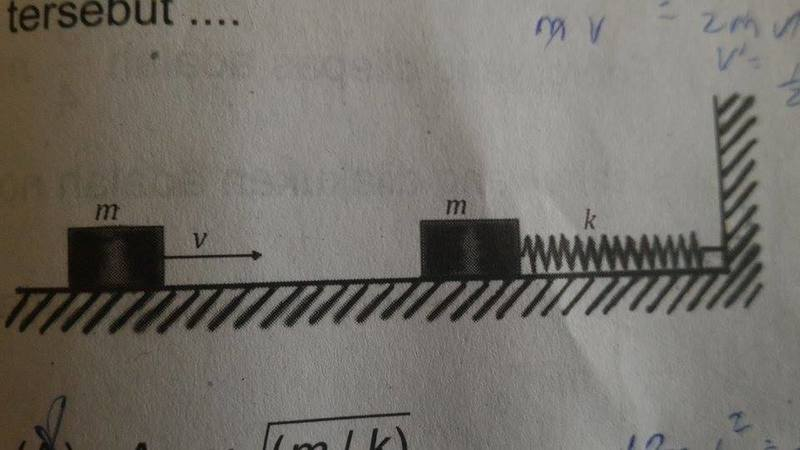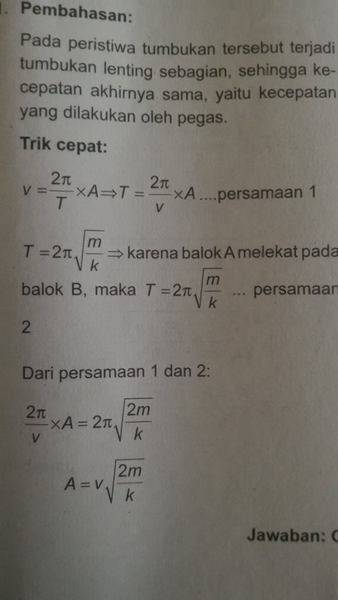# Amplitude of spring after collision

## Homework StatementA block with mass m which is on a slippery floor is attached to a spring with spring constant k.
Another block which mass is also m approach and then hit the first block with velocity v as shown in the figure.
After the collision, both blocks stick together and together oscillates on the spring.
The amplitude of the oscillation is

A. A = v √(m/k)
B. A = v √(k/m)
C. A = v √(2m/k)
D. A = v √(m/2k)
E. A = 2v √(m/k)

## Homework Equations

Momentum and energy conservation (I think)

## The Attempt at a Solution

[/B]
Since both blocks stick together, it's inelastic collision.

m v = 2m v'
v' = (1/2)v

1/2 (2m) (v')^2 = 1/2 k (Δx)^2
m (1/4) v^2 = 1/2 k (Δx)^2
Δx^2 = (1/2)v^2m/k
Δx = v √(m/2k)

So, I choose D as the answer..

But, the book says that it is Cwhich is very confusing how to get v = (2π/T) * A.. The explanation is very bad..

andrewkirk
Homework Helper
Gold Member
Your calculations look correct to me. Perhaps the book has a mistake. They sometimes do.
The formula ##v=\frac{2\pi}T\times A## gives the velocity v of an object in simple harmonic motion (SHM) when it passes the equilibrium point. It is easily derived when you consider that the formula for SHM is

$$x(t)=A\sin(2\pi t/T)$$

SHM is what will be happening after the collision, and the point of the collision is the equilibrium point, as that is where the spring is under no strain in either direction, and velocity is maximum.

Even though I don't understand much Indonesian/Malaysian, I can see what the book is doing. They use the formula ##T=2\pi\sqrt{\frac{mass}k}## for the period of an object in SHM, together with the other formula, to solve for ##A##. They correctly set ##mass=2m##. However they make a mistake with ##v##. They should use ##v'=v/2## instead, because that's what the velocity is after the collision.

So you are correct and the book made a mistake.
bagus!

Last edited:
•terryds
Your calculations look correct to me. Perhaps the book has a mistake. They sometimes do.
The formula ##v=\frac{2\pi}T\times A## gives the velocity v of an object in simple harmonic motion (SHM) when it passes the equilibrium point. It is easily derived when you consider that the formula for SHM is

$$x(t)=A\sin(2\pi t/T)$$

SHM is what will be happening after the collision, and the point of the collision is the equilibrium point, as that is where the spring is under no strain in either direction, and velocity is maximum.

Even though I don't understand much Indonesian/Malaysian, I can see what the book is doing. They use the formula ##T=2\pi\sqrt{\frac{mass}k}## for the period of an object in SHM, together with the other formula, to solve for ##A##. They correctly set ##mass=2m##. However they make a mistake with ##v##. They should use ##v'=v/2## instead, because that's what the velocity is after the collision.

So you are correct and the book made a mistake.
bagus!

Thanks a lot